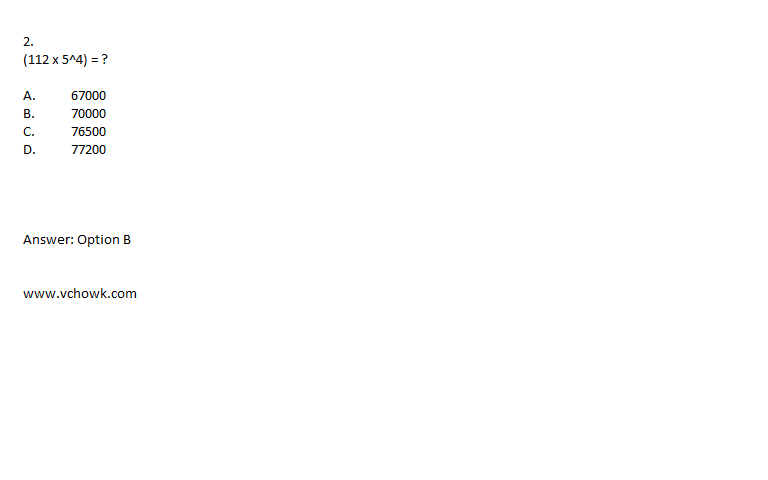Page 1 of 1

### Aptitude > Numbers > General QuestionsPosted: Wed Aug 21, 2013 2:20 pm
Aptitude > Numbers > General Questions

1.
Which one of the following is not a prime number?

A. 31
B. 61
C. 71
D. 91

Explanation:

91 is divisible by 7. So, it is not a prime number.### Re: Aptitude > Numbers > General QuestionsPosted: Wed Aug 21, 2013 2:30 pm
3.
What least number must be added to 1056, so that the sum is completely divisible by 23 ?

A. 2
B. 3
C. 18
D. 21
E. None of these

5.
1397 x 1397 = ?

A. 1951609
B. 1981709
C. 18362619
D. 2031719
E. None of these

6.
How many of the following numbers are divisible by 132 ?
264, 396, 462, 792, 968, 2178, 5184, 6336

A. 4
B. 5
C. 6
D. 7

Explanation:

132 = 4 x 3 x 11

So, if the number divisible by all the three number 4, 3 and 11, then the number is divisible by 132 also.

264 11,3,4 (/)

396 11,3,4 (/)

462 11,3 (X)

792 11,3,4 (/)

968 11,4 (X)

2178 11,3 (X)

5184 3,4 (X)

6336 11,3,4 (/)

Therefore the following numbers are divisible by 132 : 264, 396, 792 and 6336.

Required number of number = 4.

### Re: Aptitude > Numbers > General QuestionsPosted: Wed Aug 21, 2013 2:33 pm
8.
The largest 4 digit number exactly divisible by 88 is:

A. 9944
B. 9768
C. 9988
D. 8888
E. None of these

9.
Which of the following is a prime number ?

A. 33
B. 81
C. 93
D. 97

Explanation:

Clearly, 97 is a prime number.

### Re: Aptitude > Numbers > General QuestionsPosted: Sun Oct 05, 2014 1:33 pm

### Re: Aptitude > Numbers > General QuestionsPosted: Sun Oct 05, 2014 1:40 pm

### Re: Aptitude > Numbers > General QuestionsPosted: Sun Oct 05, 2014 1:41 pm
12.
The sum of first five prime numbers is:

A. 11
B. 18
C. 26
D. 28

Explanation:

Required sum = (2 + 3 + 5 + 7 + 11) = 28.

Note: 1 is not a prime number.

Definition: A prime number (or a prime) is a natural number that has exactly two distinct natural number divisors: 1 and itself.

### Re: Aptitude > Numbers > General QuestionsPosted: Sun Oct 05, 2014 1:43 pm
13.
The difference of two numbers is 1365. On dividing the larger number by the smaller, we get 6 as quotient and the 15 as remainder. What is the smaller number ?

A. 240
B. 270
C. 295
D. 360